## How to easily calculate age in excel?

Forum to talk about all the latest technologies - ins and outs!!
Admin
Site Admin
Posts: 291
Joined: Tue Sep 25, 2007 12:41 pm

### How to easily calculate age in excel?

There are several ways to calculate age in Excel.

Let's say, in the following table I will calculate the age of Jens Hendrik.

So in cell C2 enter =(YEAR(NOW())-YEAR(B2))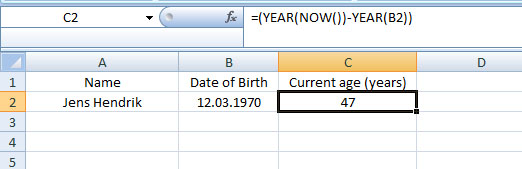Here instead of any current date, I have used the NOW function, which returns the current date and time. The function has no arguments. It's syntax is:

NOW()

So the result gives you 47 years. If you get problem with the output in cell C2, it might be because of cell format. Check the cell format and put it either on General or Number.

Second example:

In the following case, enter in cell D2 =(C2-B2)/365.25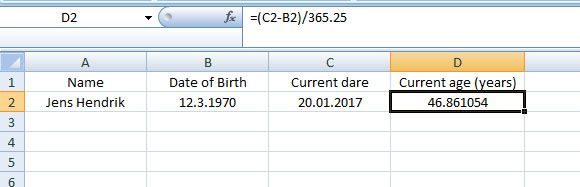Why 365.25? - because leap year occurs in every 4 years. So the answer is: 46.86 years. If you format cell D2 to Number with decimal places 0, then you get 47 years like the first example.

Third example: YEARFRAC Function

Enter in cell D2 =YEARFRAC(B2;C2)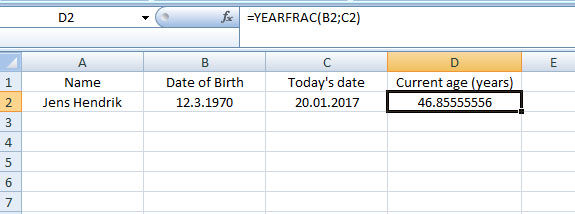The YEARFRAC function returns the fraction of a year between two dates. You get the years with a fraction - here 46.85 years. If you format cell D2 to Number with decimal places 0, then you will get 47 years.

By the way, the syntax for YEARFRAC function is

=YEARFRAC (startdate, enddate, [basis])

Fourth Example: INT function

Excel's INT function rounds a number down to the nearest integer . It's Syntax is

INT(number)

You can use the INT function several ways. Here I demonstrated only two:

Enter in cell D2 =INT((C2-B2)/365)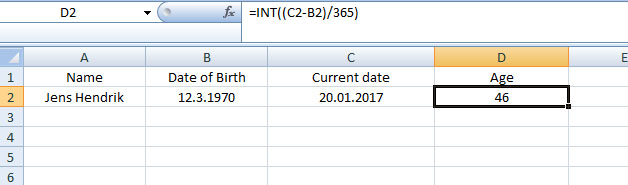So you get 46 years.

Here, in the following example, you only need the date of biirth. The TODAY() function returns you current date. So in cell C2 enter =INT((TODAY()-B2)/365)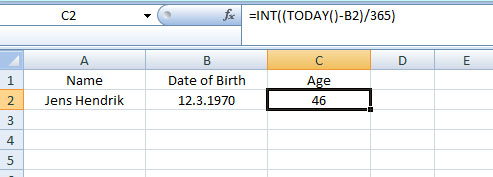Fifth Example: DATEDIF function

As you see, none of the functions above give you the exact age, means in years, months or days. For this we will use Excel's DATEDIF function.

The DATEDIF function calculates the number of days, months, or years between two dates. It's syntax is

=DATEDIF(startdate,enddate,unit).

The DATEDIF function has three arguments - startdate, enddate, and unit. Remember, in the DATEDIF function, startdate must be lower or equal to the enddate, otherwise you will get an error!! And about 'unit', you have 6 possibilities:

"Y" – returns the difference (between two dates) as full years

"M" - returns the difference (between two dates) as full months

“D” - returns the difference (between two dates) in days

“ym” - returns months between two dates but ignores years

“md” - returns days between two dates but ignores months and years

“yd” - returns days between two dates but ignores years

Let's look at the following example: I have calculated age in Column D. Next to it, in Column E, you see the DATEDIF function used in cells D2, D3 and D4 to calculate the differences in years, then months and finally in days, i.e. the difference between the two dates is 46 years or 562 months or 17116 days.

One thing, you have to put the 'unit' within double quote, like – "Y".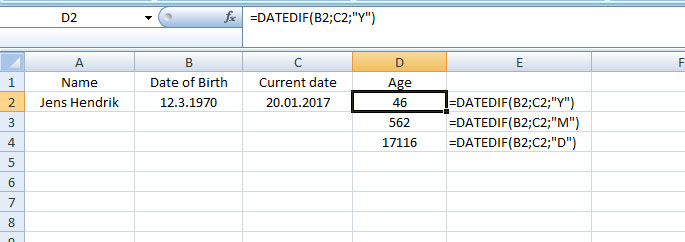But it's still not the exact answer we want. To get the accurate difference between the two dates, assuming they are more than a year apart, use the DATEDIF function as follows, enter in cell D2:

=DATEDIF(B2;C2;"Y") & " Years, " & DATEDIF(B2;C2;"ym") & " Months," & DATEDIF(B2;C2;"md") & " Days"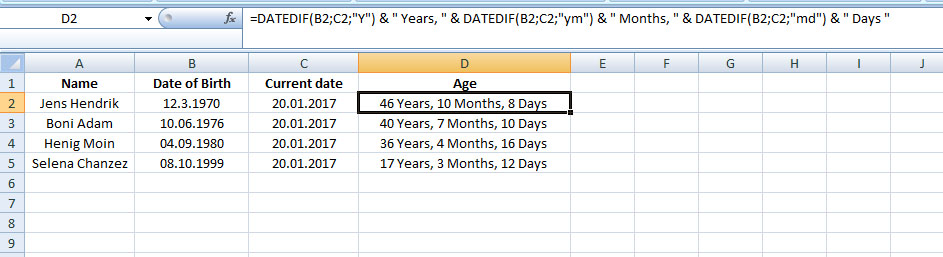So now you have the accurate age for Jens Hendrik – 46 years, 10 months and 8 days. Copy the formula down the column for the rest.

Use of TODAY() function

In the example above, under current date, I have manually entered a fixed date, which is 20.01.2017 but if you would like to enter today's date, then use TODAY() function. So it will be (in cell C2)

=DATEDIF(B2;TODAY();"Y") & " Years, " & DATEDIF(B2;TODAY();"ym") & " Months," & DATEDIF(B2;TODAY();"md") & " Days"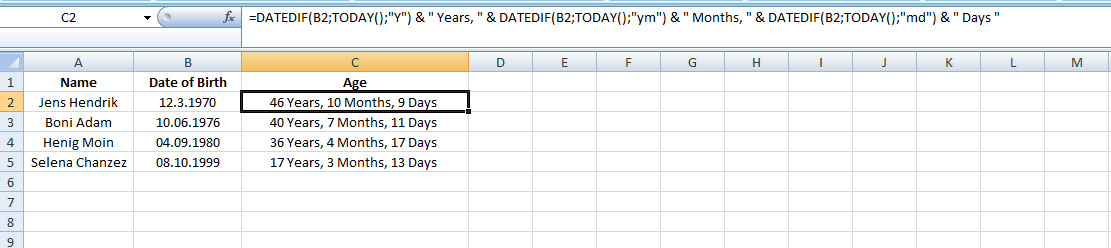So here Jens Hendrik is one day older - 46 years, 10 months and 9 days. Because as of today, it's 21.01.2017. Now copy the formula down the column for the rest.

I guess, I need a break now – coffee breakHave fun guys*Recommended:

Read wonderful articles (English & German) on Science & Tech, Environment, Health and many other topics only on BlogArena.

For comments of suggestions, please contact us: info@shamskm.com